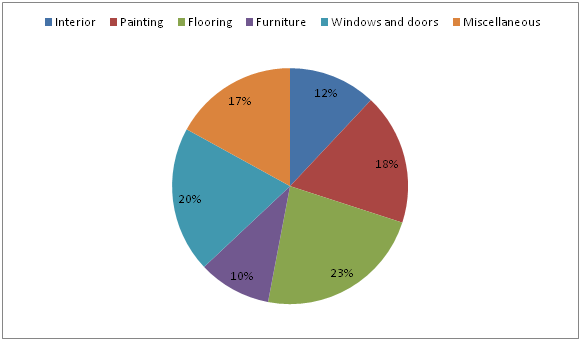# IBPS RRB Clerk Prelims Quantitative Aptitude Questions 2019 (Day-14)

Dear Aspirants, Our IBPS Guide team is providing new series of Quantitative Aptitude Questions for IBPS RRB Clerk Prelims 2019 so the aspirants can practice it on a daily basis. These questions are framed by our skilled experts after understanding your needs thoroughly. Aspirants can practice these new series questions daily to familiarize with the exact exam pattern and make your preparation effective.

### Click Here to Subscribe Crack High Level Puzzles & Seating Arrangement Questions PDF 2019 Plan

[WpProQuiz 6797]

Directions (Q. 1 – 5): What value should come in the place of question mark (?) in the following questions?

1) 24 % of 250 + ? × 4 = 6148

a) 2156

b) 2378

c) 1745

d) 1522

e) None of these

2) 43 % of 1700 – (4/7) of 5061 = ? – 133

a) 78

b) 36

c) 52

d) 64

e) None of these

3) 13310 ÷ 22 + 72 × 32 + 78 % of 5150 = ?

a) 5274

b) 5890

c) 6926

d) 6528

e) None of these

4) (9/4) of 1828 + (21/8) ÷ (63/72) =?

a) 4116

b) 5274

c) 6158

d) 6320

e) None of these

5) 15% of 440 + 22 % of 2150 = ? + √324 % of 700

a) 556

b) 625

c) 327

d) 413

e) None of these

Directions (Q. 6 – 10): Study the following information carefully and answer the given questions?

The following pie chart shows the percentage distribution of total estimated cost for renovating the house.

Total estimated cost = Rs. 5000006) If the family gets a discount on furniture and pays 15 % less than the estimated cost, then find the amount spent on furniture?

a) Rs. 41000

b) Rs. 43500

c) Rs. 42500

d) Rs. 43000

e) None of these

7) Find the average estimated cost of Interior, Flooring and Furniture together?

a) Rs. 75000

b) Rs. 72000

c) Rs. 78000

d) Rs. 74000

e) None of these

8) Find the ratio of the estimated amount of Interior and painting together to that of the estimated amount of Windows and doors and miscellaneous together?

a) 14 : 19

b) 5 : 7

c) 23 : 29

d) 30 : 37

e) None of these

9) The estimated amount of painting is what percentage more/less than the estimated amount of windows and doors?

a) 10 % more

b) 10 % less

c) 15 % less

d) 15 % more

e) None of these

10) Out of the total estimated cost of furniture, 54 % for showcase, then find the difference between the estimated cost for showcase to that of the estimated cost for cab board and shelf’s in a room?

a) Rs. 4000

b) Rs. 3500

c) Rs. 4250

d) Rs. 4800

e) None of these

Direction (1-5) :

(24/100) * 250 + 4x = 6148

60 + 4x = 6148

4x = 6148 – 60

4x = 6088

x = 1522

(43/100) * 1700 – (4/7) * 5061 = x – 2197

731 – 2892 + 2197 = x

x = 36

(13310/22) + (72*32) + (78/100)*5150 = x

x = 605 + 2304 + 4017

x = 6926

(9/4) * 1828 + (21/8) * (72/63) = x

x = 4113 + 3

x = 4116

(15/100) * 440 + (22/100) * 2150 = x + (18/100) * 700

66 + 473 = x + 126

66 + 473 – 126 = x

x = 413

Direction (6-10) :

Total estimated cost on furniture = 500000 * (10/100) = Rs. 50000

Discount = 15 %

So, the amount spent on furniture = 500000 * (85/100) = Rs. 42500

The average estimated cost of Interior, Flooring and Furniture together

= > 500000 * [(12 + 23 + 10) / 100] * (1/3)

= > Rs. 75000

The estimated amount of Interior and painting together

= > [(12 + 18) / 100] * 500000

The estimated amount of Windows and doors and miscellaneous together

= > [(20 + 17) / 100] * 500000

Required ratio = 30 : 37

The estimated amount of painting = 500000 * (18/100)

The estimated amount of windows and doors = 500000 * (20/100)

Required % = [(20 – 18) / 20] * 100 = 10 % less

Required difference

= > 500000 * (10/100) * (54/100) – 500000 * (10/100) * (46/100)

= > 500000 * (10/100) * [(54 – 46) / 100]

= > 500000 * (10/100) * (8/100)

= > Rs. 4000